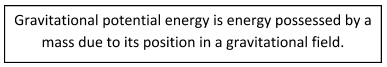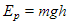# Conservation Of Energy

## Introduction

Consider a scenario: When diesel is burned in a truck engine, some of the chemical energy stored in the form of liquid is converted into kinetic energy of the truck and some are wasted as thermal energy. When the truck stops, its kinetic energy is converted into internal energy in the brakes. The temperature of the brakes increases and heat energy is released. The outcome is that chemical energy has been converted into heat energy, which dissipates in the atmosphere and is of no further use. However, the total energy present in the universe has remained constant.

A. Principle of Conservation of Energy

All energy changes are governed by the law of conservation of energy. The law states that energy cannot be created or destroyed. It can only be converted from one form to another.

B. Kinetic Energy

Kinetic energy is associated with moving objects. As we know, a moving object can be made to do work as it slows down. For example, a moving hammer hits a nail and, as it stops, it does work to drive the nail into a wall.

The kinetic energy Ek joules (J) of an object of mass m (kg) moving with speed v (m/s) is given by the following equation. Ek is termed as translational kinetic energy since it is energy due to an object moving in a straight line. Rotating objects also have kinetic energy and that is known as a rotational form of energy.C. Potential Energy

Newton’s law of gravitation tells us that all masses attract one another. When two masses are pulled apart, work is done on them and so they gain gravitational potential energy. If the masses move closer together, they lose gravitational potential energy.If an object of mass m (kg) moves a vertical distance of h (m), then the gravitational potential energy stored in the object is defined by the following equation:Consider a wagon that moves at a velocity reaching the top of a hill. From the top, it reaches the ground level. Figure 1 explains kinetic and potential energy of the wagon at three different places. At ground level, the wagon does not have potential energy. At the top of the hill, the wagon does not move. At that point, the potential energy of the wagon is at its highest and the kinetic energy of the wagon is zero.Figure 1: Kinetic and Potential Energy

D. Applications of Conservation of Energy

The law of conservation of energy is a fundamental concept that is used in several scientific fields. Concepts such as kinetic energy and gravitational potential energy are used in designing cranes, elevators and roller-coaster rides.

Example 1

A librarian stacks a bookshelf with 22 books, each with a mass of 350 g. Each book has to be raised by 2.2 m. Calculate the gravitational potential energy gained by the books.

Total mass raised = 22 x 350 = 7700 g = 7.7 kg

Increase in potential energy, Ep=m × g × h

= 7.7 x 9.8 x 2.2

= 166 J

Example 2E. Summary

• Energy cannot be created or destroyed. It can only be converted from one form to another.
• Kinetic energy is energy due to motion.
• Gravitational potential energy is energy possessed by a mass due to its position in a gravitational field.